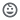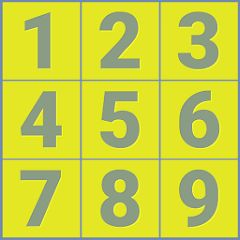# ninedigits workshop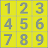10K+Everyone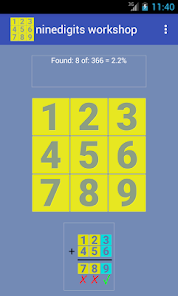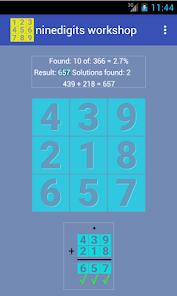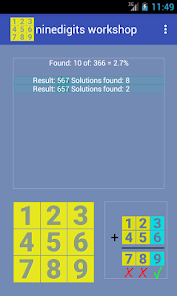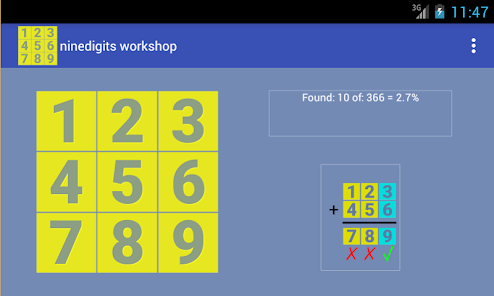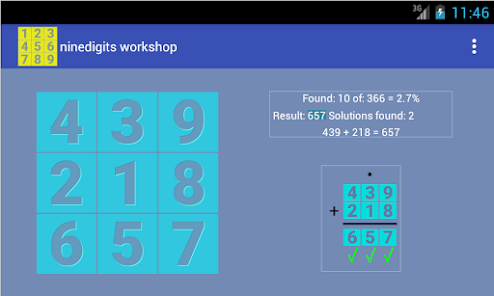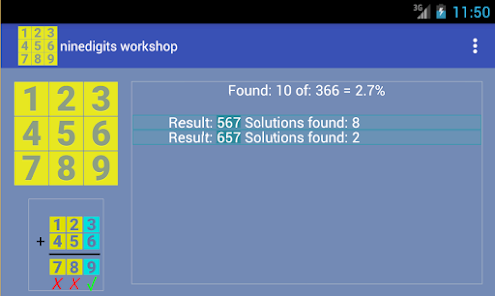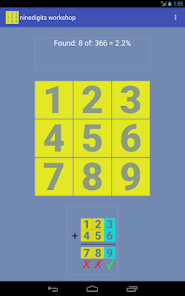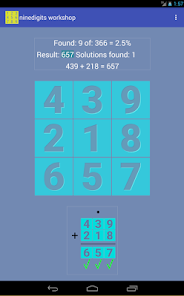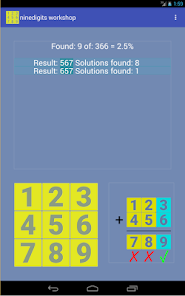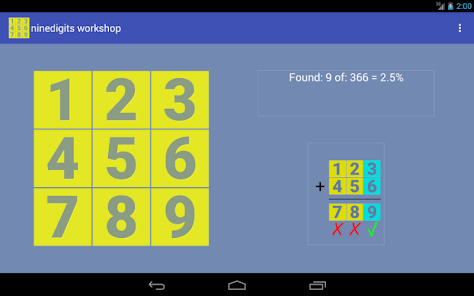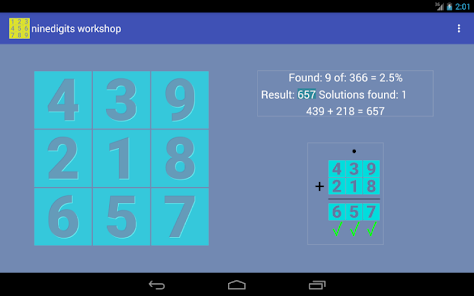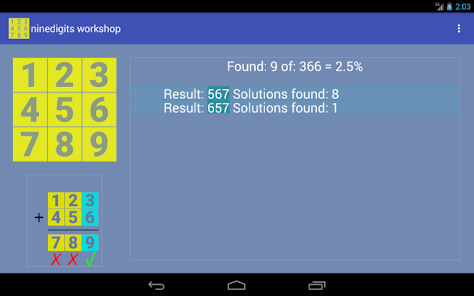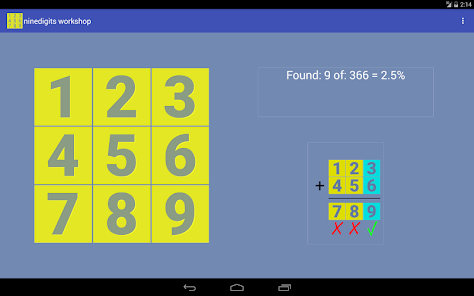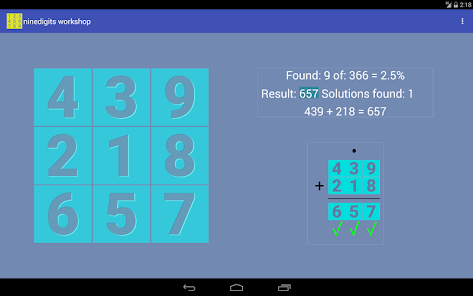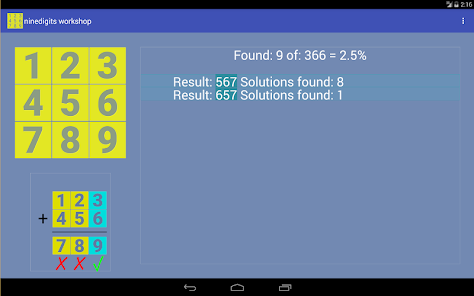The game is about to find solutions sorting the digits from 1 to 9 in a square of 3 * 3 where the sum of the numbers of the two top rows is equal to the bottom row.

This puzzle is aimed on reflection on the commutative property of addition.

This program is aimed at reflecting on the addition. The goal is to find results that meet the primary condition. We must be aware that after obtaining a correct result can be achieved more easily results having in mind the properties of the sum.

Interaction:
To swap two digits must be done tap on each digit, then the digits change the color, and the exchange occurs.

From:
http://www.nummolt.com/obbl/ninedigits/ninedigitsbasic.html
nummolt - Obbl - Math Toys collection - Mathcats.

Ninedigits has 336 solutions. If the program would be easy for someone, then the goal could be to find valid solutions in which a Queen (Lady) could travel chess boxes 1 to 9 making right moves to this tab. According to our analysis, there are 3 solutions of this type. You can also look under the same condition, but with the Tower (Rock) of chess. This combination of conditions has only one solution. The program clearly shows the production of these special results.

As a safety mechanism, the delete button only works when the program displays a correct solution of the problem.

Registered in Math Tools (MathForum):
http://mathforum.org/mathtools/tool/234619/
Classified for courses:
Math 5 Addition, Mental math, Commutative
Math 6 Addition, Mental math, Commutative
Math 7 Mental math, Commutative

Aligned with Common Core Math:

Grade 3 » Number & Operations in Base Ten
CCSS.Math.Content.3.NBT.A.2
Fluently add and subtract within 1000 using strategies and algorithms based on place value, properties of operations, and/or the relationship between addition and subtraction.

Origin of the game:
The ninedigits is based on an new idea described in a Martin Gardner's. mathematical book of diversions: published in 1966.

Nine Digits and the chain of numbers problem:
All the right results involve an addition of 3 digits with trading.

To get results quickly you have to reflect on module 9 of each line.
The third line, the result line, will always be MOD 9= 0
And the sum of the MOD 9 of each the first two lines will also be 0.

Nummolt apps: Math Garden: Prime Numbers Barn and Numbers Mill
Updated on
Nov 25, 2023

## Data safety

Safety starts with understanding how developers collect and share your data. Data privacy and security practices may vary based on your use, region, and age. The developer provided this information and may update it over time.No data shared with third parties